# Study on Sloshing of Liquid Tank in Large LNG-FSRU Based on CLSVOF Method

Study on Sloshing of Liquid Tank in Large LNG-FSRU Based on CLSVOF Method

Wang Chong Qin Hongde* Liu Ge Guo Ting

College of Shipbuilding Engineering, Harbin Engineering University, Harbin 150001, China

School of Naval Architecture, Dalian University of Technology, Dalian 116024, China

Corresponding Author Email:
qinhongde@hrbeu.edu.cn
Page:
616-622
|
DOI:
https://doi.org/10.18280/ijht.340410
|
Accepted:
|
Published:
31 December 2016
| Citation

OPEN ACCESS

Abstract:

In the light of the advantages and disadvantages of the level-set method and VOF method, a new coupling method of the liquid interface tracing named CLSVOF (Coupled Level-Set and Volume-of-Fluid method) has been proposed in recent years. By means of this method, this paper realizes the conservation of physical quantities in the calculation process compared with the level-set method, and overcomes the difficulty of accurately calculating the interface normal and curvature in comparison with the VOF method. Specifically, the above method is utilized in this paper to numerically simulate the sloshing of a liquid tank and its results are compared with the experimental results. It is found that the results agree with each other, which verifies the validity and accuracy of the CLSVOF method. Afterwards, this paper takes the liquid cargo tank of a large LNG-FSRU (Liquefied Natural Gas-Floating Storage Regasification Unit) as its research object to conduct a three-dimensional numerical simulation of rolling motion and study the influence of the excitation centre on the sloshing of the liquid tank. It turns out that the position of the excitation centre affects the movement of the free surface and the pressure distribution of the tank. Meanwhile, research on the position of the excitation centre provides the theoretical foundation for designing the marine structure of the octagonal tank.

Keywords:

Level-set method, Volume-of-fluid method, CLSVOF method, Large LNG-FSRU, Excitation centre.

1. Introduction

The sloshing of the liquid tank is a common phenomenon. In terms of a ship, the liquid cargo tank fixed to the hull moves along with the motion of the hull. In this condition, there is a free surface inside the tank when the liquid cargo tank is partially loaded, therefore the liquid inside the tank would move due to the excitation of the hull movement, which refers to the sloshing of the liquid cargo tank. In recent years, there has been an increase in research on the sloshing of the liquid tank. Under these circumstances, the research methods of liquid tank sloshing contain theoretical research, numerical calculation, experimental research, and so forth. However, the theoretical research is faced with the difficulty of solving partial differential equations, and the experimental means suffer from the problem of a long cycle and high cost. With the rapid development of computer technology, numerical calculation has become the main means to study the sloshing of liquid tanks, and there are various methods available for its application [1-4].

One common difficulty encountered in the numerical method analysis of the liquid sloshing is how to accurately determine the position of the free surface, which refers to the tracking of the moving interface. The existing tracking methods can be broadly divided into two categories, namely the front capturing and the front tracking. Specifically, the front capturing methods include the continuous transport method, VOF method [5-6], level-set method [7-8], and phase field method. Meanwhile, the front tracking methods include the moving grid method, MAC method, wave height function method, and particle method. The level-set method and the VOF method both utilize one scalar function to describe geometric characteristics of the interface, which easily deals with the topological changes of the interface. Thus, these two methods have gained relatively wide attention and application. However, after in-depth investigation, the level-set method and VOF method both have obvious strengths and weaknesses. In this regard, the level-set method can implicitly track the interface, and the level-set function is always smooth regardless of the change of the flow field, which is liable to deal with the complex interface deformation or topology changes. Nevertheless, as a non-conservative method, there is a loss of physical quantity in the calculation process and the screeding phenomenon of the sharp interface in the level-set method. Although the conservation of physical quantity can be well guaranteed with the VOF method, it is hard to calculate the normal and curvature of the interface accurately and extend them to a high dimension due to the complicated method of interface reconstruction. Hence, it is essential to seek a new method to combine the advantages of the level-set method and the VOF method and overcome the above-mentioned disadvantages.

To address the above problems, a coupling method of the interface tracking named CLSVOF has been used in recent years . The CLSVOF method takes advantage of the level-set function to calculate the volume fraction of VOF, which overcomes the shortcomings of the VOF method in accurately calculating the normal and curvature of the interface. Meanwhile, the level-set function is modified by using the volume fraction of VOF as the level-set method is not a conservative method which has a loss of physical quantity in the calculation process. In this paper, the coupling realization of the CLSVOF method is expatiated, and the validity and accuracy of the CLSVOF method in solving practical engineering problems are verified by numerical experiments. Afterwards, the sloshing of liquid tanks in large LNG-FSRU based on the CLSVOF method is studied in order to obtain the influence of different excitation centres on the liquid tank sloshing of the double-tank structure.

2. Numerical Method

2.1 Mathematical method

It is assumed that the fluid in the tank with variable properties is incompressible and shows constraint continuity. In terms of the standard κ-ε turbulence model, the fluid motion restricted to the constraint continuity can be controlled by Reynolds-averaged Navier-Stokes (RANS) equations [10-11].

$\frac{\partial \rho}{\partial \mathrm{t}}+\frac{\partial}{\partial x_{i}}\left(\rho u_{i}\right)=0$(1)

$\frac{\partial}{\partial \mathrm{t}}\left(\rho u_{i}\right)+\frac{\partial}{\partial x_{j}}\left(\rho u_{i} u_{j}\right)=-\frac{\partial p}{\partial x_{i}}+\frac{\partial}{\partial x_{j}}\left[\mu\left(\frac{\partial u_{i}}{\partial x_{j}}+\frac{\partial u_{j}}{\partial x_{i}}-\frac{2}{3} \delta_{i j} \frac{\partial u_{l}}{\partial x_{l}}\right)\right]+\frac{\partial}{\partial x_{j}}\left(-\rho \overline{u_{l}^{\prime} u_{j}^{\prime}}\right)$(2)

where $u_{i}, u_{j}$ and $u_{l}$$(i, j, l=1,2,3) are the time average velocity, x_{i} and x_{j} are the coordinates in longitudinal, transverse and vertical directions, respectively, p is the time average pressure, \rho is the fluid density, \mu is the kinetic viscosity of water, \delta_{i j} is the Kronecker delta, and -\rho \overline{u_{\imath}^{\prime} u_{\jmath}^{\prime}} is the Reynolds stress term. Regarding the standard k-\varepsilon turbulence model, the turbulence kinetic energy k and its rate of dissipation, \mathcal{E} , can be obtained based on the following transport equations: \frac{\partial}{\partial \mathrm{t}}(\rho k)+\frac{\partial}{\partial x_{j}}\left(\rho k u_{i}\right)=\frac{\partial}{\partial x_{j}}\left[\left(\mu+\frac{\mu_{l}}{\sigma_{k}}\right) \frac{\partial k}{\partial x_{j}}\right]+G_{k}+G_{b}-\rho \varepsilon-Y_{M}+S_{k}(3) \frac{\partial}{\partial \mathrm{t}}(\rho \varepsilon)+\frac{\partial}{\partial x_{i}}\left(\rho \varepsilon u_{i}\right)=$$\frac{\partial}{\partial x_{j}}\left[\left(\mu+\frac{\mu_{l}}{\sigma_{\varepsilon}}\right) \frac{\partial \varepsilon}{\partial x_{j}}\right]+C_{1 \varepsilon} \frac{\varepsilon}{k}\left(G_{k}+C_{3 \varepsilon} G_{b}\right)-C_{2 \varepsilon} \rho \frac{\varepsilon^{2}}{k}+S_{\varepsilon}$ (4)

In the above equations, $G_{k}$  represents the generation of turbulence kinetic energy due to the mean velocity gradients. $G_{b}$  is the generation of turbulence kinetic energy due to buoyancy. $Y_{M}$  represents the contribution of the fluctuating dilatation in compressible turbulence to the overall dissipation rate. $C_{1 \varepsilon}, C_{2 \varepsilon},$ and $C_{3 \varepsilon}$  are constants. $\sigma_{k}$ and $\sigma_{\varepsilon}$  are the turbulent Prandtl numbers for $k$ and $\varepsilon$ , respectively. $S_{k}$ and $S_{\varepsilon}$  are user-defined source terms.

The turbulent (or eddy) viscosity, $\mu_{t}$ , can be calculated using the following equation:

$\mu_{t}=\rho C_{\mu} \frac{k^{2}}{\varepsilon}$(5)

The default values of the constants $C_{1 \varepsilon}, C_{2 \varepsilon}, C_{\mu}, \sigma_{k}$ and $\sigma_{\varepsilon}$  in the model are as follows:

$C_{1 \varepsilon}=1.44, C_{2 \varepsilon}=1.92, C_{\mu}=0.09, \sigma_{k}=1.0 \sigma_{\varepsilon}=1.3$

2.2 Level-set method

Regarding the level-set method, the time-varying material interface is treated as the zero-isosurface of a function $\varphi(\mathrm{x}, \mathrm{t})$ so that the moving interface $\Gamma(t)$ is exactly the zero-isosurface of the LS function as below at any time.

$\Gamma(\mathrm{t})=\{\mathrm{x} \in \Omega | \varphi(\mathrm{x}, \mathrm{t})=0\}$(6)

where $\Omega$  is the fluid region; $\mathrm{x}$ is the vector coordinate.

The initial value of $\varphi$  should be a continuous function in the vicinity of $\Gamma(0)$  and zero on $\Gamma(0) ; \varphi(\mathrm{x}, \mathrm{t})$ represents the signed distance function from point $\mathrm{x}$  to interface $\Gamma(0)$ , which is

$\varphi(x, 0)\left\{\begin{array}{c}{d[x, \Gamma(0)] x \in \Omega_{1}} \\ {0 \quad x \in \Gamma(0)} \\ {-d[x, \Gamma(0)] x \in \Omega_{2}}\end{array}\right.$(7)

where $d[x, \Gamma(0)]$ indicates the distance function from point $\mathrm{X}$  to interface $\Gamma(0) ; \Omega_{1}$ and $\Omega_{2}$  represent the area where the first and second kind of medium are located respectively.

At any time $(\mathrm{t}), \quad \varphi(\mathrm{x}, \mathrm{t})=0$ should be guaranteed in regard to any point x on the moving interface $\Gamma(\mathrm{t})$ . Thus the function $\varphi(\mathrm{x}, \mathrm{t})=0$ should satisfy the following condition:

$\frac{\partial \varphi}{\partial \mathrm{t}}+\nabla \cdot(\mathrm{U} \varphi)=0$(8)

The calculation for the normal vector ( $n$ ) and the curvature ( $k$ ) of the moving interface $\Gamma(t)$ could be obtained using the following equation:

$\mathrm{n}=\frac{\nabla \varphi}{|\nabla \varphi|} \cdot k=\nabla \cdot \frac{\nabla \varphi}{|\nabla \varphi|}$(9)

Since the numerical calculation process is error prone, the calculated $\varphi(\mathrm{x}, \mathrm{t})$  based on equation (8) may no longer be the distance from point $\mathrm{x}$  to the interface at time $t$ , and the zero-isosurface may be inequivalent to the moving interface leading to computational instability. Consequently, for the sake of maintaining the properties of the signed distance function, $\varphi(\mathrm{x}, \mathrm{t})$ should be re-initialized for becoming the signed function from point x to interface $\Gamma(t)$ again. Meanwhile, the re-initialization process would be implemented by solving the following initial value problem:

$\left\{\begin{array}{c}{\varphi_{\eta}=\operatorname{sign}\left(\varphi_{u}\right)(1-|\nabla \varphi|)} \\ {\varphi(x, 0)=\varphi_{u}}\end{array}\right.$(10)

where $\eta$  is the virtual iteration time.

2.3 VOF method

In terms of the VOF method, $F(\Omega, t)$ is used to express the volume fraction of the fluid occupied in each computational grid unit after defining one volume function $F(\Omega, t)$ ( $\Omega$  is the computational grid unit). The volume function $F(\Omega, t)$ is described in the examples of gaseous medium and liquid medium as shown below:

if $\left\{\begin{array}{c}{F(\Omega, t)=1 \text { reference phase }} \\ {F(\Omega, t)=0 \text { other phase }} \\ {0<F(\Omega, t)<1 \text { free surface }}\end{array}\right.$(11)

Obviously, $\Omega$ contains a moving interface when $0<F(\Omega, t)<1$ , so the grid unit of the moving interface can be determined by solving the volume function $F(\Omega, t)$ ;. Since the value of the function $F(\Omega, t)$ changes most rapidly along the normal direction of the moving interface, the derivative value of the function $F(\Omega, t)$  determines the direction and position of the moving interface.

The governing equation of the VOF method is as follows:

$\frac{\partial F}{\partial t}+(V \cdot \nabla) F=0$(12)

Where $V$  is the speed.

2.4 CLSVOF method

The VOF volume function $F(\Omega, t)$ is defined by the level-set function $\varphi(x, y, t)$ so as to couple the level-set function with the VOF volume function. Let $\varphi>0$  in the liquid region and $\varphi<0$  in the gas region, thus

$\mathrm{F}(\Omega, \mathrm{t})=\frac{1}{|\Omega|} \int_{\Omega} H(\varphi(x, y, t)) d x d y$(13)

where $H$  represents the Heaviside function.

$H(\varphi)=\left\{\begin{array}{cc}{1} & {\text {if } \varphi>0} \\ {0} & {\text { otherwise }}\end{array}\right.$(14)

Owing to the non-smooth Heaviside function in equation(14), it is easy to cause the loss of physical conserved quantity in the process of numerical calculation. Therefore, this paper corrects the equation (14) and introduces the smooth Heaviside function :

$H(\varphi)=\left\{\begin{array}{cc}{0,} & {\varphi<-a} \\ {\frac{1}{2}\left[1+\frac{\varphi}{a}-\frac{1}{\pi} \sin \left(\frac{\pi \varphi}{a}\right)\right],} & {|\varphi| \leq a} \\ {1,} & {\varphi>a}\end{array}\right.$(15)

where a is the interface thickness.

The density and viscosity after smoothing is

$\rho(\varphi)=\rho_{g}+\left(\rho_{l}-\rho_{g}\right) H(\varphi)$(16)

$\mu(\varphi)=\mu_{g}+\left(\mu_{l}-\mu_{g}\right) H(\varphi)$(17)

In equations(16) and (17), $\rho$ and $\mu$ denote the density and dynamic viscosity respectively; $g$ and $l$  represent the gas phase and liquid phase separately.

The two-phase flow control equation is:

$\nabla \cdot u=0$

$\rho(\varphi)\left(\frac{\partial u}{\partial t}+u \cdot \nabla u\right)=-\nabla p+\rho(\varphi) g-\sigma k(\varphi) \nabla H(\varphi)+\nabla \cdot \tau$(18)

$\tau=2 \mu(\varphi) S$

where $u$  is the speed; $\boldsymbol{p}$  is the intensity of pressure; $g$ is the acceleration of gravity; $\sigma$ is the surface tension coefficient; $\tau$  is the viscous stress tensor; $S$  is the strain-rate tensor; $\sigma k(\varphi) \nabla H(\varphi)$ is the surface tension term, which is coupled to the momentum equation as a volume force with reference to Brackbill ; $\delta(\varphi)=\frac{1+\cos \left(\frac{\pi \varphi}{a}\right)}{2 a}$ if $|\varphi|<a$ and $a=1.5 h$ (where $h$  is the grid spacing), otherwise $\delta(\varphi)=0$ .

In this paper, the PISO algorithm is adopted to solve the pressure- velocity coupling equation, and the discretization of the convective term and the level-set equation is conducted in the QUICK scheme. Besides, the diffusion terms are obtained through the interpolation of the least squares method based on the unit volume, and the pressure term is in the PRESTO format. Meanwhile, the second-order upwind scheme is adopted for the energy equation.

3. Comparison of Numerical Results of CLSVOF Method

## 1.png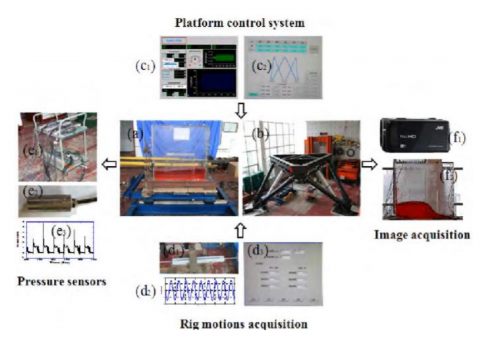Figure 1. Sloshing model test system

In order to verify whether the CLSVOF method is capable of simulating the liquid tank sloshing well, the results given in this paper should be compared with the experimental results of WEI Zhi-jun , and WEI's sloshing model test system is illustrated in Figure 1. In the experiment, the size of the rectangular liquid tank is selected as shown in Figure 2, which has an inner wall with a length of 970 mm (L), a width of 158 mm (B), and a height of 927 mm (H). At a low carrier liquid rate (h/H=0.2), the nonlinearity of the fluid-free surface is stronger and its physical phenomenon is more complex. When the exciting frequency approaches the lowest-order natural frequency of the free surface ( $\omega_{1}$ ), the liquid sloshing inside the tank is the most violent.

The specific experimental conditions are as follows:

The liquid inside the tank is water;

The carrier liquid rate is h/H=0.2;

The corresponding swaying excitation amplitude at this liquid level is A=30mm;

The lowest-order natural frequency is chosen as the exciting frequency, which refers to f1=0.660 Hz.

## 2.png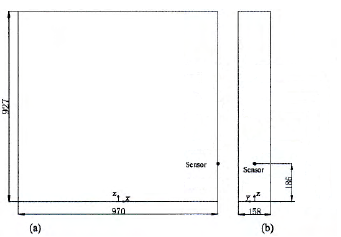Figure 2. Tank geometry and pressure monitoring point configuration (mm): (a) Front view; (b) Side view

It can be seen from Figure 3 that the numerical simulation results obtained from the FLUENT software and the experimental results accord with each other, which verifies the accuracy of the CLSVOF method. Meanwhile, Figure 4 illustrates the comparison of the liquid sloshing phenomenon and the experimental phenomenon, which proves that the addition of the moving items on the basis of the FLUENT software could simulate the sloshing phenomenon of the liquid tank well.

## 3.png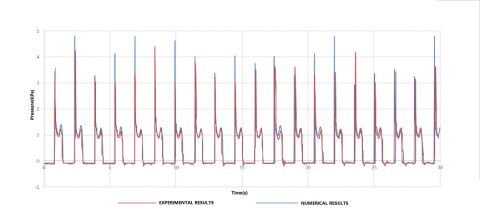Figure 3. Comparison between the experimental and numerical results of pressure at the monitoring point

## 4.png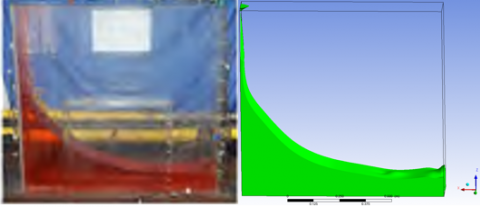Figure 4. Comparison between the experimental and     numerical results of the liquid sloshing

4. The Impact of The Excitation Centre Position on The Liquid Sloshing of LNG-FSRU

In the single-row-tank LNG vessel, its excitation centre is located on the centerline along the longitudinal direction while studying the rolling of the tank. However, regarding the double-row-tank LNG-FSRU shown in Figure 5 , its excitation centre lies on the centerline of the hull instead of the liquid cargo tank. In the case of the tank rolling of a LNG-FSRU, the CLSVOF method is applied to simulate the influence of the excitation centre in different positions on the tank rolling numerically.

## 5.png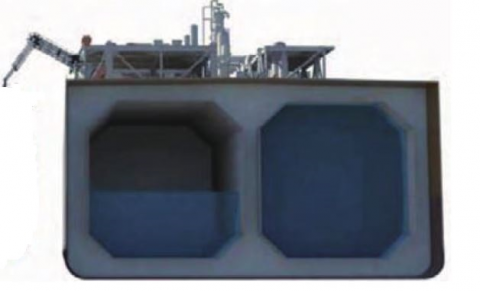Figure 5. Schematic diagram of the liquid tank in LNG-FSRU

4.1 Numerical simulation

The typical GTT membrane tank is exhibited in Figure 5, and 68.7% of the current storage tank adopts this type of prismatic tank, according to some relevant investigation . In addition, a numerical model is established for the liquid tank of a LNG-FSRU with the geometric model shown in Figure 6and initial section size in Table 1.

The simulating parameters are as follows:

The liquid inside the tank is liquefied natural gas (LNG);

The carrier liquid rate is h/H=0.3;

The corresponding swaying excitation amplitude at this liquid level is $\theta_{1}$ 0.071rad;

The lowest-order natural frequency is chosen as the exciting frequency, which refers to f2=0.161 Hz.

## 6.png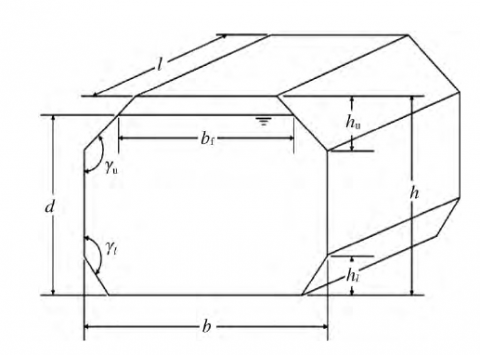Figure 6. Geometric model of the tank

Table 1. Inner dimensions of model tank

 Parameter Value $\boldsymbol{l} / \mathbf{m}$ 54.236 $\mathbf{b} / \mathbf{m}$ 28.024 $\mathbf{h} / \mathbf{m}$ 24.024 $\mathbf{h}_{l} / \mathbf{m}$ 4.839 $\mathbf{h}_{\boldsymbol{u}} / \mathbf{m}$ 4.839 $\gamma_{l}, \gamma_{u} /\left(^{\circ}\right)$ 135

For the purpose of better comparison, this paper selects the carrier liquid rate of 30% (h/H=0.3) for simulation to calculate the rolling of the liquid tank with an excitation centre in four different positions, which is illustrated in Figure 7. In particular, the actual excitation centre of the tank is displayed in the working condition A, and the geometric centre of the tank is shown in the working condition B.

The 3D numerical model of the liquid tank was meshed in the pre-processing program (ICEM CFD), which is demonstrated in Figure 8. The 3D model adopts 123, 200 hexahedral cells. Besides, the dynamic mesh technique is applied in the solution process. Given the velocity of the wall in UDF, the amplitude of the motion increases gradually and reaches the maximum at 50s. Moreover, the variable step size is utilized to adjust the step size by the Courant number, and the iteration time is 120 seconds.

## 7.png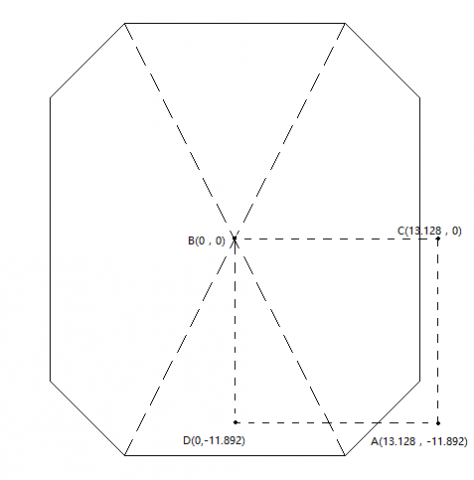Figure 7. Coordinates of the excitation centre

## 8.png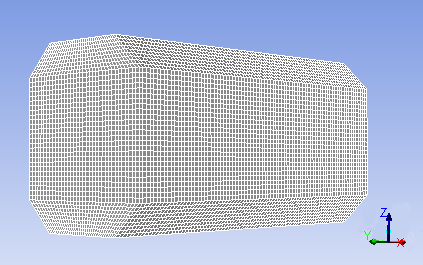Figure 8. Meshing of the numerical model of liquid tank

The above numerical models have been obtained by studying the influence of the grid number, turbulence parameter, algorithm advantage, and time step on the calculation results. In this condition, the models do not only meet the requirements of calculation accuracy, but also save calculation time.

4.2 Results and analysis of the numerical calculation

4.2.1 Simulation results for the waveform of free surfaces

By simulating the tank sloshing in four working conditions, the results of the free surface waveform shown in Figures 9and 10 are obtained. Specifically, the free surface lashes against the left-side bulkhead of the side shell in Figure 9 as well as the right-side middle bulkhead in Figure 10. From the simulation results of the conditions B and C, it can be seen that the tank sloshing is quite violent; the liquid lashes upward to a certain height along with the following natural fallback rather than rolling down. In addition, the initial wave appears to be a standing wave with a relatively long wavelength (the travelling wave is not obvious), and the ordered periodic motion of the liquid is presented along the cross-sectional direction of the tank in the whole moving process. As seen from the simulation results of the conditions A and D, the liquid tank sloshing is more violent than that in conditions B and C, which shows a significant impact of the liquid on the left and right sides of the bulkhead. The liquid can continue to climb along the bulkhead after lashing against the bulkhead; nevertheless, the phenomenon of the liquid impacting the top is not formed owing to the relatively small excitation amplitude. During the whole motion, the waveform of the interface shape is the typical travelling wave. At the same time, the simulated results in conditions A and C are left-right asymmetric, and the results in Figure 9 are significantly stronger than those in Figure 10, which is due to the fact that the excitation centre is outside the tank in the transverse direction.

## 9.png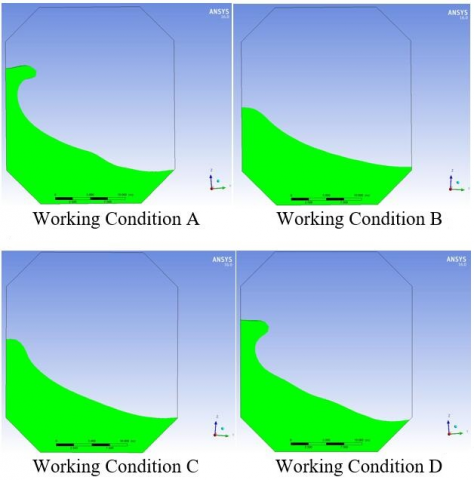Figure 9. The free surface lashes against the left-side bulkhead of the side shell

## 10.png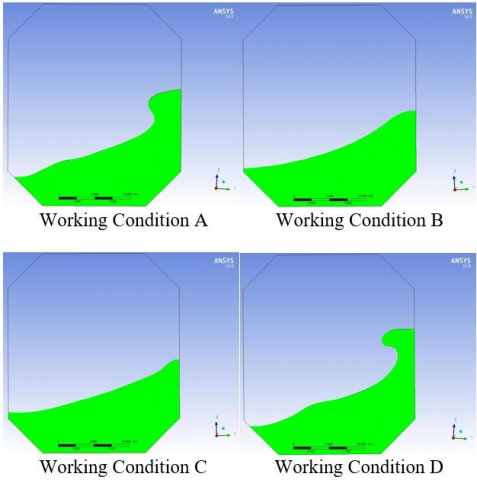Figure 10.The free surface lashes against the right-side middle bulkhead

4.2.2 Simulation results of the pressure at the monitoring point near the free surface

By setting the pressure monitoring point at the left and right free surfaces, the pressure change curve of the monitoring point at the free liquid surface is demonstrated in Figures 11(a), (b), (c), (d). As shown, there is strong nonlinearity in the results of the conditions A and D. The pressure on the left and right sides of conditions A and C differs, and the pressure away from the excitation centre is greater than that close to the excitation centre. The extreme values of the conditions A and D exceed those of the conditions B and C.

The above results are analyzed below.

(1) The position of the excitation centre has a significant impact on the sloshing of the liquid tank. The lower the position of the excitation centre, the greater the pressure on the free surface caused by the sloshing.

(2) When the excitation centre in the cross-sectional direction is located outside the structure, it gives rise to uneven stressing on the left and right sides, which means that the pressure away from the excitation centre is greater than that close to the excitation centre.

(3) As a comparison between the conditions A and D shows, the double-rowtank structure can reduce the pressure of the tank sloshing on the structure.

## 11a.png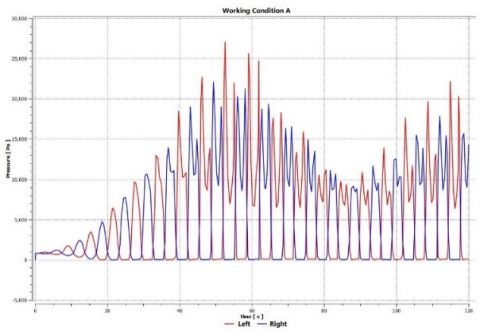(a)Working Condition A

## 11b.png(b)Working Condition B

## 11c.png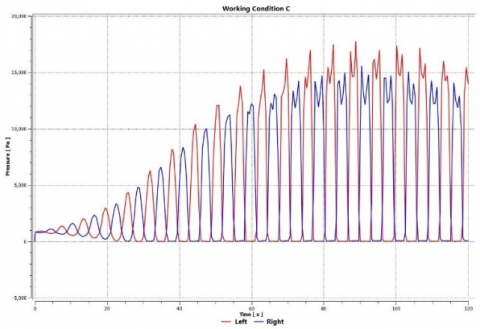(c)Working Condition C

## 11d.png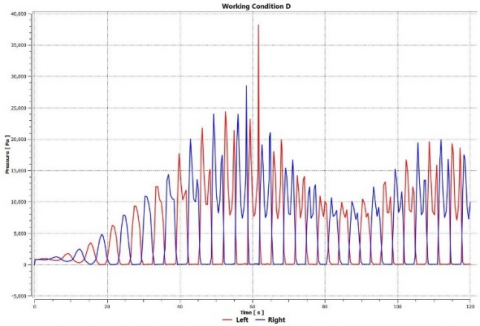(d)Working Condition D

Figure 11. The change curve of pressure at the monitoring point on the free surfaces (a), (b), (c), (d)

5. Conclusions

This paper elaborates the generation process of the coupling method of the interface-CLSVOF, and the numerical simulation of the rectangular tank sloshing by means of the Fluent software platform is compared with the experimental results. Hence, it is verified that the CLSVOF method utilizes the level-set function to calculate the volume fraction of VOF, and the VOF method is applied to correct the physical conservation of the level-set method. Furthermore, the numerical simulation of a membrane-type cargo tank of a LNG-FSRU is conducted in the CLSVOF method to obtain the free surface waveform with the excitation centre in different positions and results of pressure on the free surface. Therefore, the main conclusions are as follows:

1. The CLSVOF method is capable of tracking the fluid interface accurately, which is used for more precise simulation of liquid tank sloshing.
2. The sloshing load increases with the decrease of the excitation centre position, and the nonlinear phenomenon of the liquid surface movement becomes more obvious. When the excitation centre is outside the tank, the influence of the tank sloshing on the structure would weaken under the same condition.
3. Research on the excitation centre demonstratesthat the position of the excitation centre significantly affects the tank rolling, which provides the theoretical foundation for the design of the octagonal tank structure.
References

 Lu Yu, Hu An-kang, Liu Ya-chong, “Application of Finite Point Method to Liquid Sloshing in Tanks,” Journal of South China University of Technology (Natural Science Edition), vol. 43, no.8, pp. 144-150, 2015. DOI: 10.3969/j.issn.1000-565X.2015.08.021.

 Armenio V., “An improved MAC method ( SIMAC) for un-steady high-reynolds free surface flows,” Interna-tional Journal for Numerical Methods in Fluids, vol. 24, no. 2, pp. 185-214, 1998. DOI: 10.1002/(SICI)1097-0363 (19970130)24:2<185::AIDFLD487>3.0.CO;2-Q.

 Kim Y., “Numerical simulation of sloshing flows with impact load,” Applied Ocean Research, vol. 23, no. 1, pp. 53-62, 2001. DOI: 10.1016/S0141-1187(00)00021-3.

 T. Cardinale, P. de Fazio and F. Grandizio, “Numerical and experimental computation of airflow in a transport container,” International Journal of Heat and Technology, vol. 34, Special Issue 2, pp. S323-S331, 2016. DOI: 10.18280/ijht.34S219.

 Sethian J.A., “Fast marching methods,” SIAM Review, vol. 41, no. 2, pp. 199-235, 1999. DOI: 10.1137/S0036144598347059.

 Osher S., Sethian J.A., “Fronts propagating with curvature dependent speed: algorithms based on Hamilton Jacobi formulation,” Journal of Computational Physics, vol. 79, no. 1, pp. 12-49, 1988. DOI: 10.1016/0021-9991(88)90002-2.

 Hirt C.W., Nichols B.D., “Volume of Fluid (VOF) method for the dynamics of free boundary,” Journal of Computational Physics, vol. 39, no. 1, pp. 201-225, 1981. DOI: 10.1016/0021-9991(81)90145-5.

 Li C.M., Xu C.Y., Gui C.F., et al., “Level set evolution without re-initialization: a new variational formulation,” IEEE CVPR, vol. 1, no. 1, pp. 430-436, 2005. DOI: 10.1109/CVPR.2005.213.

 Liao B., Chen S.Q., “Interface tracking coupled based on CLSVOF Method,” Periodical of Ocean University of China, vol. 43, no. 9, pp. 106-111, 2013.

 Lv Suiju and Feng Minquan, “Three-dimensional numerical simulation of flow in Daliushu reach of the Yellow River,” International Journal of Heat and Technology, vol. 33, no. 1, pp. 107-114, 2015. DOI: 10.18280/ijht.330115.

 Cai Zhong-Hua, Wang De-Yu, Li Zhe, “Experimental study and numerical simulation of sloshing in VLGC tanks with internal structures,” Journal of Ship Mechanics, vol. 16, no. 12, pp. 1385-1393, 2012.

 Ménard T., Tanguy S., Berlemont A., “Coupling level set/VOF/ghost fluid methods: Validation and application to 3D simulation of the primary break-up of a liquid jet,” International Journal of Multiphase Flow, vol. 33, no. 5, pp. 510-524, 2007. DOI:10.1016/j.ijmultiphaseflow.2006.11.001.

 Brackbill J.U., Kothe D.B., Zemach C., “A Continuum method for modeling surface tension,” Journal of Computational Physics, vol. 100, no. 2, pp. 335-354, 1992. DOI: 10.1016/0021-9991(92)90240-Y.

 Wei Zhi-Jun, Chen Xiao-Dong, Dong Yu-Shan, et al., “An experimental study of slamming impact load on two platforms,” Journal of Ship Mechanics, vol. 19, no. 7, pp. 841-849, 2015. DOI: 10.3969/j.issn.1007-7294.2015.07.010.

 Chen Shi-wen, Yang Zhi-xun, Ruan Shi-lun, et al., “Dimension sensitivity analysis of octagon tank on sloshing-induced slamming load based on simulation,” Ocean Engineering Equipent and Technology, vol. 3, no.1, pp. 39-45, 2016.

 Zhu Xiao-Song, Xie Bin, Yu Xi-Chong, “Research progress of liquid sloshing in LNG/LPG tanks,” Shipbuilding  of  China, vol. 54, no.1, pp. 229-236, 2013.